# Part 4.3Loops

Loops are a crucial part of any program. A program such as a graphics program uses a loop (or multiple loops) to perform certain effects.

The two loops are used in the previous example and are used for:

1. One loop for each column of the image
2. One loop inside the first loop for each row of the image

This means that every y is performed for every single x. So for an image of 400 pixels wide by 300 pixels high, the program performs precisely 120,000 operations. That means for every one of the 400 pixels, it does 300 operations.

Loops are not just limited to graphics applications however. For instance, counting numbers 1 to 1000 and placing them on the screen. Loops are designed to save time. When a loop is set to repeat something x number of times, each step is called an iteration.

In VB.NET there are several loops:

• While
• Do
• For
• For Each

## While loop

A while loop is a loop that continues while a condition is true. A while loop starts with the word While and ends with End While. It looks something like the following sample:

VB.NET
While condition
'Place code to be repeated here
End While


The following are simple examples of while statements.

VB.NET
Dim i As Integer = 0
While i < 100
Dim m As Integer
m = i * 3
Console.Write(m)
i+=1
End While


The previous example outputs all numbers from 0 to 99 multiplied by 3.

VB.NET
Public Module FindNumber

Sub Main()
FindNumberByIndex(16)
End Sub

Public Sub FindNumberByIndex(ByVal index As Integer)
Dim i As Integer = 0
While i < 100
Dim m As Integer
m = MathsWork(i)
If i = index Then
MsgBox(m)
End If
i+=1
End While
End Sub

Public Function MathsWork(ByVal n As Integer) As Integer
Return n * 3
End Function

End Module


The previous example is used to return the value of a number given to some function that, in this case, just multiplies by three.

## Do loop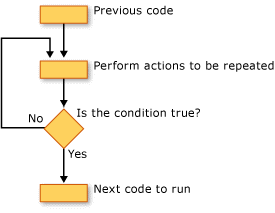A do loop continues to do something until a condition is met. It is composed of two to three keywords, of which two are absolutely required known as the essentials of a do loop. These are Do and Loop.

VB.NET
Do [Keyword] [condition]
'Place code to be repeated here
Loop


Both the keyword and condition are optional as shown in the previous example. This mean a loop does not need the second keyword and by removing it, the loop will go forever. It can however be terminated, as this article will discuss further on. The following example is an example of an infinite loop.

VB.NET
Dim i As Integer
Do
i+=1
MsgBox(i)
Loop


The optional keywords that can be used to terminate when something happens are:

• While
• Until

A Do While...Loop is used to perform a task while some condition is true. A Do Until...Loop is used to perform a condition while some condition is not true (or until some condition is true).

VB.NET
Dim i As Integer
Do Until i > 10
i+=1
MsgBox(i)
Loop


The previous example is an example of a loop that ends when it reaches 11 (a number greater than 10). Using the Until keyword, the loop keeps working until it reaches 11.

VB.NET
Dim i As Integer
Do While i < 11
i+=1
MsgBox(i)
Loop


This loop performs the same function but instead of using Until it uses While. This program however, must now compare the opposite way so instead of the > (greater than) 10 condition, it must now say less than 11. The choice of these loops can be very much a personal choice.

Do loops can also be inverted, meaning the condition comes last. The following are some samples that are the same as the previous two examples but inverted.

These kinds of loops will always run the code inside the loop at least once because the condition is checked last.

VB.NET
Dim i As Integer
Do
i+=1
MsgBox(i)
Loop Until i > 10

VB.NET
Dim i As Integer
Do
i+=1
MsgBox(i)
Loop While i < 11


## For loop

There are two types of for loop, but they are generally seen as two seperate loops. The first for loop is used to create a very simple looping structure. It saves the creation of a variable as it is created within the statement and disposed of when the loop is complete. A for loop has the advantage of defining a starting point from within the loop itself. There are three essentials of a For Loop; namely For , To and Next .

The following demonstrates the basic structure of a for loop:

VB.NET
For declaration = start To end [Step by]
'Place code to be repeated here
Next


The declaration placeholder is where the variable declaration goes, the start placeholder is where the number to start the loop goes and the end placeholder is where the end value will go (where the loop will terminate) and the optional Step parameter is used to define how much to increment by each time.

If a for loop goes from 0 to 9 and the step parameter is set to 4, the 9 will be skipped and the for loop will end.

The following is an example of a standard for loop.

VB.NET
For position As Integer = 0 To 30
Console.WriteLine(position)
Next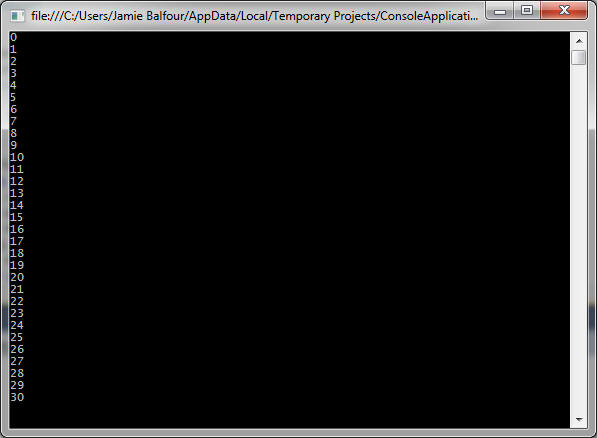The following example uses the step parameter

VB.NET
For position As Integer = 0 To 30 Step 5
Console.WriteLine(position)
Next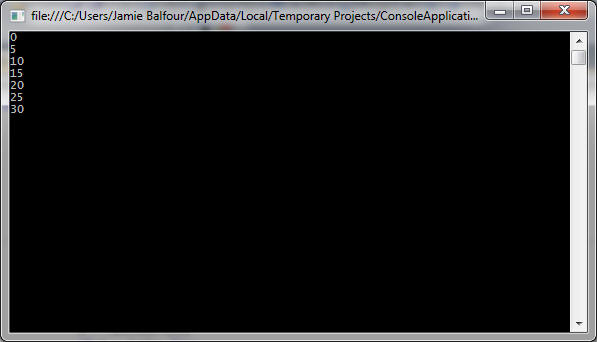The previous example uses the step parameter to jump by 5 rather than 1, incrementing the value of position by 5.

The next example shows populating an array of 10 items using a for loop.

VB.NET
Dim names(10) As String
For i As Integer = 0 To 10
Next


## For each loop

In some other languages that implement the for each loop, it is simply represented as a for loop. VB.NET makes it easier to decipher a for loop from a for each loop.

A for each loop is designed to iterate through a collection (a programming term for more than object) and automatically offer each object as if it were a variable. The next example shows the basic structure of a for each loop.

VB.NET
For Each [declaration] In collection
'Place code to be repeated here
Next


The following user interface is used for the sample following it.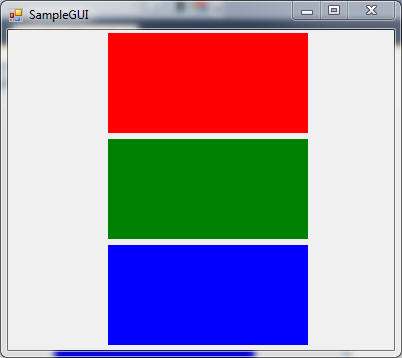VB.NET
For Each control As Control In Me.Controls
MsgBox(control.BackColor.ToString())
Next


The following images are the output message boxes for the above sample.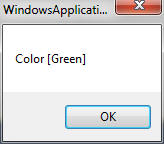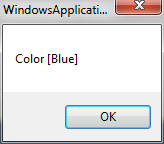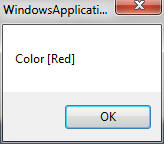Another example that is a bit more useful is one that will iterate an array of strings:

VB.NET
Dim words(2) As String
words(0) = "Hello"
words(1) = "There"
words(2) = "World"

For Each str As String In words
MsgBox(str)
Next


A for each loop can be considered a bit more robust than a for loop but more limited.

Feedback 👍
Comments are sent via email to me.